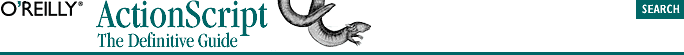home | O'Reilly's CD bookshelfs | FreeBSD | Linux | Cisco | Cisco Exam## 5.9. The Comma Operator

`operand1, operand2`

Both operands must be legal expressions. When a comma operation executes, operand1 is evaluated, then operand2 is evaluated, and then the resolved value of operand2 is returned. In practice, the return value of the comma operator is usually ignored.

The comma operator is primarily used in for loops that initialize multiple variables as shown in Example 5-6. Note that in the first line of the example, the expressions i=0 and j=10 are both operands of the comma operator. (These expressions cause i and j to be initialized to and 10.) The expressions i++ and j-- are both operands of a second comma operator. (These expressions cause i to be incremented and j to be decremented each time the loop executes.) The comma operator is used in order to squeeze multiple expressions within the confines of the for loop's syntax.

#### Example 5-6. Using the Comma Operator in a for Loop

```for (var i=0, j=10; i!=j; i++, j--) {
trace ("i: " + i + " j: " + j);
}

// Which produces this output...
i: 0 j: 10
i: 1 j: 9
i: 2 j: 8
i: 3 j: 7
i: 4 j: 6```